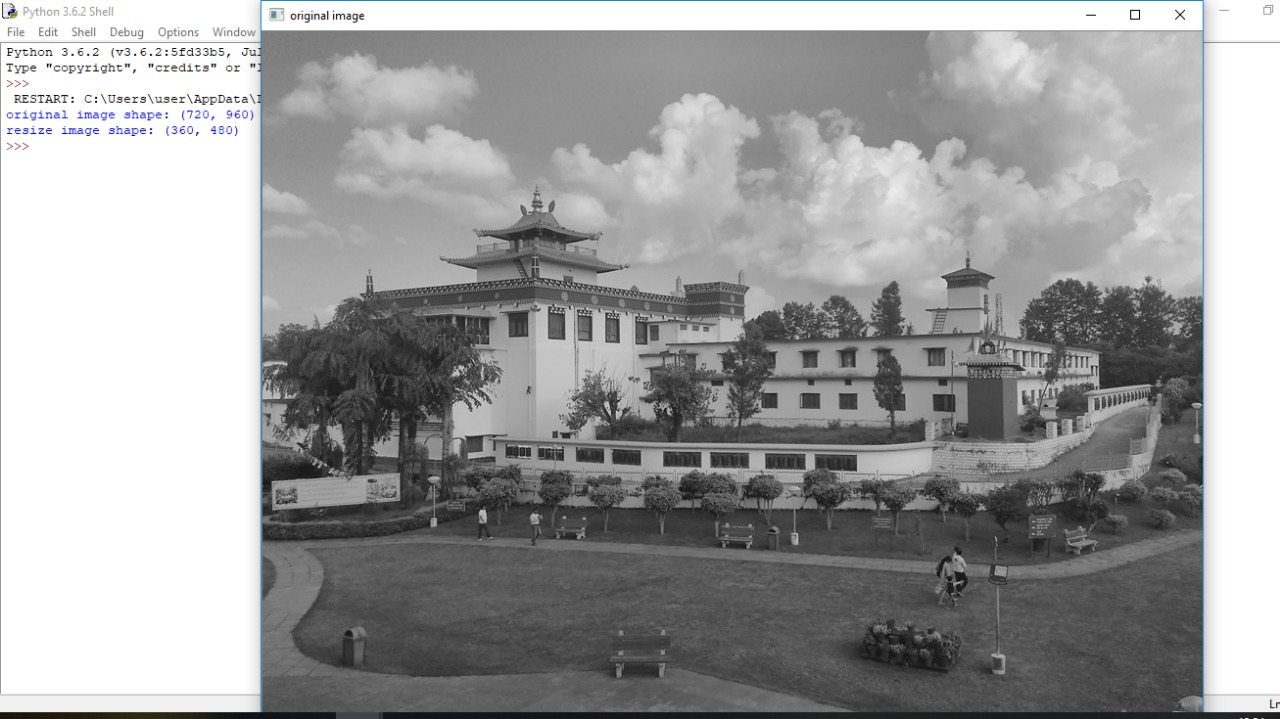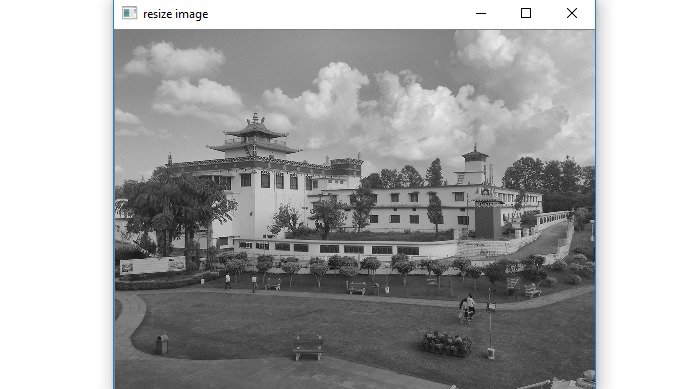# Resize a grayscale image without using any inbuilt functions in Python

In this article, we will see how to resize a gray scale image without using any inbuilt function?
Submitted by Ankit Rai, on May 01, 2019

In this program, we will be using two functions of OpenCV-python (cv2) module. Let's see their syntax and descriptions first.

It takes an absolute path/relative path of your image file as an argument and returns its corresponding image matrix.

2) imshow():
It takes window name and image matrix as an argument in order to display an image in a display window with a specified window name.

Also In this program, we will be using one attribute of an image matrix:

shape: This is the attribute of an image matrix which return shape of an image i.e. consisting of number of rows ,columns and number of planes.

In the case of a Grayscale image, only one plane is needed. If the number of planes is 1 then shape attribute only return number of rows and columns.

Also, here we are using the concept of array slicing

Let, A is 1-d array:
A[start:stop:step]

1. start: Starting number of the sequence.
2. stop: Generate numbers up to, but not including this number.
3. step: Difference between each number in the sequence.

Example:

```    A = [1,2,3,4,5,6,7,8,9,10]
print(A[ 1 : : 2])

Output:
[2, 4, 6, 9]
```

### Python program to resize a grayscale image without using any inbuilt functions

```# open-cv library is installed as cv2 in python
# import cv2 library into this program
import cv2

# give value by which you want to resize an image
# here we want to resize an image as one half of the original image
x,y= 2,2

# we have to  pass only the path of the image

# displaying the image using imshow() function of cv2
# In this : 1st argument is name of the frame
# 2nd argument is the image matrix
cv2.imshow('original image',img)

# print shape of the image matrix
# using shape attribute
print("original image shape:",img.shape)

# here we take alternate row,column pixel.
# we take half pixel of rows and columns respectively
# so that it is one half of image matrix.
resize_img = img[::x,::y]

cv2.imshow('resize image',resize_img)

# print shape of the image matrix
# using shape attribute
print("resize image shape:",resize_img.shape)
```

OutputPreparation